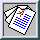Physics of Fluids 6 (1994) pp. 1036-1051

### Similarity states of passive scalar transport in isotropic turbulence

J. R. Chasnov
Center for Turbulence Research, NASA Ames Research Center

We determine the time-evolution of the kinetic energy and scalar-variance in decaying isotropic turbulence transporting passive scalars using simple analytical arguments and large-eddy simulations. The evolution of a passive scalar field with and without a uniform mean gradient is considered. Firstly, we consider similarity states of the flow during the final period of decay. Exact analytical solutions may be obtained and these depend only on the form of the energy and scalar-variance spectra at low wavenumbers, and the molecular transport coefficients. The solutions for a passive scalar field with mean-scalar gradient are of special interest and we find that the scalar-variance may grow or decay asymptotically in the final period depending on the initial velocity distribution. Secondly, we consider similarity states of the flow at high Reynolds and Peclet numbers. Here we assume that the solutions also depend on the low wavenumber spectral coefficients, but not on the molecular transport coefficients. This results in a non-linear dependence of the kinetic energy and scalar-variance on the spectral coefficients, in contrast to the final period results. The analytical results obtained may be exact when the similarity solutions depend only on spectral coefficients which are time-invariant. Our analysis also leads directly to a similarity state for a passive scalar field with uniform mean scalar gradient. Lastly, we perform large-eddy simulations of the flow field to test the theoretical results. Asymptotic similarity states at large times in the simulations are obtained and found to be in good agreement with predictions of the analysis. We also determine several dimensionless quantities which compare favorably to earlier experimental results. An argument for the inertial subrange scaling of the scalar-flux spectrum is presented which yields a spectrum proportional to the scalar gradient and decaying as k-7/3. This result is partially supported by the small scale statistics of the large-eddy simulations.

Full Text: [PDF (2.1 MB) GZipped PS(1.8 MB)]